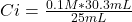## A 25.0mL solution of KOH is neutralized with 30.3 mL of 0.100M HBr. What is the concentration of the original KOH solution?

Question

A 25.0mL solution of KOH is neutralized with 30.3 mL of 0.100M HBr. What is the concentration of the original KOH solution?

in progress 0
5 months 2021-08-13T16:51:35+00:00 1 Answers 6 views 0

## Answers ( )

The concentration of the original KOH solution is 0.1212 M.

Explanation:

Dilution is the process to reduce the concentration of solute in solution. This is accomplished by adding more solvent to the same amount of solute. In other words, dilution is the procedure followed to prepare a less concentrated solution from a more concentrated one, and it simply consists of adding solvent to an existing solution.

As only solvent is being added, the consequence is that by not increasing the amount of solute, but if the amount of solvent, the concentration of the solute decreases.

The expression for the dilution calculation is:

Ci* Vi = Cf* Vf

where:

• Ci: initial concentration
• Vi: initial volume
• Cf: final concentration
• Vf: final volume

In this case:

• Ci= ?
• Vi: 25 mL
• Cf: 0.1 M
• Vf: 30.3 mL

Replacing:

Ci* 25 mL= 0.1 M* 30.3 mL

Solving:Ci=0.1212 M

The concentration of the original KOH solution is 0.1212 M.